# Abel differential equation

(diff) ← Older revision | Latest revision (diff) | Newer revision → (diff)

The ordinary differential equation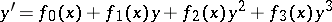(Abel's differential equation of the first kind) or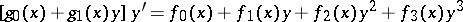(Abel's differential equation of the second kind). These equations arose in the context of the studies of N.H. Abel  on the theory of elliptic functions. Abel's differential equations of the first kind represent a natural generalization of the Riccati equation.

If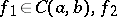andand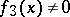for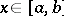, then Abel's differential equation of the first kind can be reduced to the normal form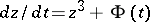by substitution of variables . In the general case, Abel's differential equation of the first kind cannot be integrated in closed form, though this is possible in special cases . If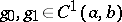and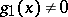,, Abel's differential equation of the second kind can be reduced to Abel's differential equation of the first kind by substituting.

Abel's differential equations of the first and second kinds, as well as their further generalizations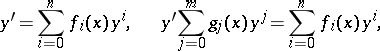have been studied in detail in the complex domain (see, for example, ).

How to Cite This Entry:
Abel differential equation. Encyclopedia of Mathematics. URL: http://encyclopediaofmath.org/index.php?title=Abel_differential_equation&oldid=18925
This article was adapted from an original article by N.Kh. Rozov (originator), which appeared in Encyclopedia of Mathematics - ISBN 1402006098. See original article